### How to Solve Syllogism Questions [Methods Explained]If you are preparing for bank exams, understanding the basic concepts on solving a particular questions is very important.Of all the sections, reasoning & quantitative aptitude are the two areas that most candidates feel tough to crack.Therefore we thought of explaining few topics that form part of the question paper regularly.Lets start it with an article on How to solve syllogism questions.

Most bank exams have at least 3-5 questions dedicated to the syllogism. If one knows the right approach, this can be your high scoring section.

Here, we will look at solving Syllogism using the Venn Diagram approach along with a few solved examples.

### What are Syllogisms

A syllogism is a kind of logical argument that applies deductive reasoning to arrive at a conclusion based on two or more premises (also called propositions) that are asserted or assumed to be true.

The conclusion asserts no more than what is already contained, implicitly, in the premises. If it does, the syllogism is invalid.

An important thing to be kept in mind is that one has to take the given statements to be true even if they seem to be at variance from commonly known facts.

### Types of Premises

A premise is a statement which comprises of two terms; a subject and a predicate, connected by a relation.

• Subject is that about which something is said and
• Predicate states something about the subject.

If another premise contains one of these terms (known as the common term), we can deduce a relation between the non-common terms.

There are four types of premises.

All A are B

The general representation of this premise is as shown below: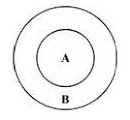But there may be a case where A=B, then also this premise is true.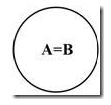No A are B

This premise is represented as: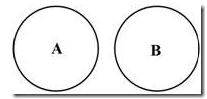Some A are B

It can be depicted as: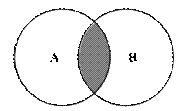The shaded portion denotes the premise.

NOTE: Looking at this general representation, one may perceive that if ‘some A are B’ then ‘some A are not B’.

But the following cases can also imply ‘Some A are B’:

- All B are A.

- All A are B.

- A and B are identical.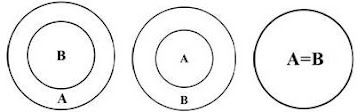We can see that in the case where

(i) A is a subset of B or

(ii) A=B,

the premise ‘some A are not B’ does not hold true.

Some A are not B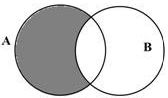This premise can also be represented as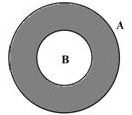Such questions could be part of the new SBI PO prelims exam & you may click here to take a free mock test at Oliveboard.You may also consider reading the review of their online mock tests

### Solving Syllogisms using Venn diagram

Venn diagram method is an effective and precise method to solve syllogism problems.You can follow the below steps to answer them:

• In order to solve a syllogism, first draw the standard diagram based on the given statements.
• Then try to check which of the given conclusions follow in every possible case.
• If a conclusion is true for one case but is negated for the other possible representation, it is not considered as a conclusion.
• In other words, a conclusion follows only if it is true for all the possible cases.

Now we shall look into some examples of solving syllogisms by Venn diagram method which will help understand the topic better.

Example 1:

Statements:

Some reds are crows.

All crows are yellows.

All yellows are rabbits.

Conclusions:

I. All crows are rabbits.

II. Some yellows are reds.

III. Some reds are rabbits.

Solution:

From the first statement, some reds are crows. This can be represented as: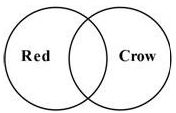Next statement says all crows are yellows. So the circle ‘yellow’ must engulf ‘crow’.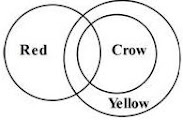Next we have all yellows are rabbits. So the circle ‘rabbit’ engulfs ‘yellow’.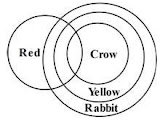This is the standard representation of all the statements taken together.Now we shall check the conclusions based on this representation.

Conclusion I: All crows are rabbits

From the diagram, we can see that the circle ‘rabbit’ engulfs the circle ‘crow’. So conclusion I follows.

Conclusion II: Some yellows are reds

We can see that the circles ‘yellow’ and ‘red’ intersect each other. So conclusion II also follows.

Conclusion III: Some reds are rabbits

We can see that the circles ‘rabbit’ and ‘red’ intersect each other. So conclusion III also follows.

Hence all of the given conclusions follow.

 Mock Tests for Practice: To improve your speed & time in the upcoming SBI PO prelims, you need to practice such questions.Checkout SBI PO Online Mock Tests (20 sets with detailed answers) – Rs.499 – Buy Online

Example 2:

Statements:

All cars are bikes

Some bikes are ships

All ships are planes

Conclusions:

I. At least some planes are bikes.

II. Some bikes are cars.

III. All planes are cars is a possibility.

Solution:

From the given statements, the standard diagram can be drawn as: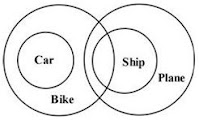From this, we can say that conclusions I and II follow, but III does not.

But we can have another representation of the given statements as below: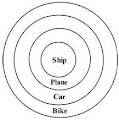Here all conclusions follow.

Conclusion III says all planes are cars is a possibility, it does not mean that it has to be definitely true. So, even if we have a single case where all planes are car, the conclusion follows.

Hence all the conclusions follow.

NOTE:

1. The conclusion ‘At least some A are B’ is true if ‘some A are B’ or ‘all A are B’.

2. A conclusion containing words like ‘possible’ or ‘possibility’ is true if it holds true for any of the possible scenarios.

If there is one such conclusion, try all the possible cases and check if any of the case the conclusion is true.

Example 3:

Statements:

Some cameras are laptops.

Some laptops are phones.

Some phones are tablets.

Conclusions:

I. At least some tablets are cameras

II. There is a possibility that all tablets are laptops

III. No tablet is a laptop

Solution:

From the given statements, the standard diagram can be drawn as: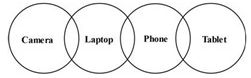According to this diagram, conclusion III follows.

But there’s a conclusion with the word ‘possibility’.So we’ll check if any such case is possible.

In that case, the diagram would look like: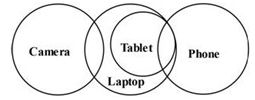But in this case, conclusion III does not follow.

Thus either conclusion II or conclusion III follows.Thats it.!!

We hope the diagrams & explanations might help you remember the methods involved to solve syllogism questions during the exams.

Oliveboard is an exam preparation site for Bank PO exams. It provides Videos, Study Material, Practice Tests and Test Series for SBI PO (Prelims & Mains) and IBPS PO (Prelims & Mains). It was selected as one of the top education startups of India.Click here to visit their site.

Get FREE Email Alerts on Bank Jobs/Exams & other Govt Recruitment news, Tips to Prepare & Books ->